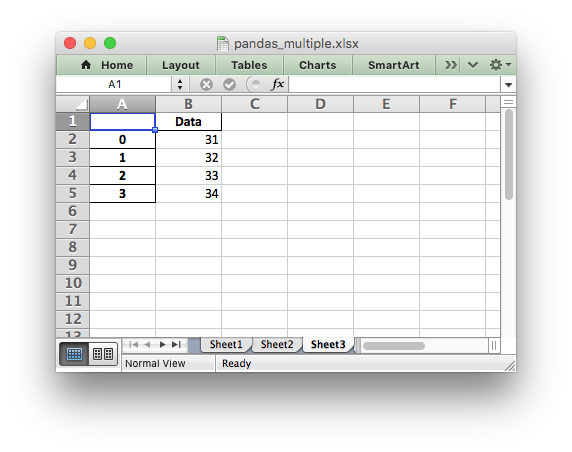# Example: Pandas Excel with multiple dataframes

An example of writing multiple dataframes to worksheets using Pandas and XlsxWriter.```##############################################################################
#
# An example of writing multiple dataframes to worksheets using Pandas and
# XlsxWriter.
#
# Copyright 2013-2023, John McNamara, jmcnamara@cpan.org
#

import pandas as pd

# Create some Pandas dataframes from some data.
df1 = pd.DataFrame({"Data": [11, 12, 13, 14]})
df2 = pd.DataFrame({"Data": [21, 22, 23, 24]})
df3 = pd.DataFrame({"Data": [31, 32, 33, 34]})

# Create a Pandas Excel writer using XlsxWriter as the engine.
writer = pd.ExcelWriter("pandas_multiple.xlsx", engine="xlsxwriter")

# Write each dataframe to a different worksheet.
df1.to_excel(writer, sheet_name="Sheet1")
df2.to_excel(writer, sheet_name="Sheet2")
df3.to_excel(writer, sheet_name="Sheet3")

# Close the Pandas Excel writer and output the Excel file.
writer.close()
```